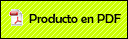# A Study of the Central Field Problem by Using the Landau-Lifshitz Equation of Motion for a Charged Particle in Classical Electrodynamics

###Ortiz Domínguez, Martín2010

Ares, G., Mares, R., Ortiz, M., (2010), A study of the central field problem by using the Landau-Lifshitz equation of motion for a charged particle in classical Electrodynamics. Journal of Vectorial Relativity 31310, 27

Abstract

The Landau-Lifshitz equation of motion for a charged particle is studied in the case of the central field problem. By using a new representation of the equation, a set of three equations are found in order to solve the problem.